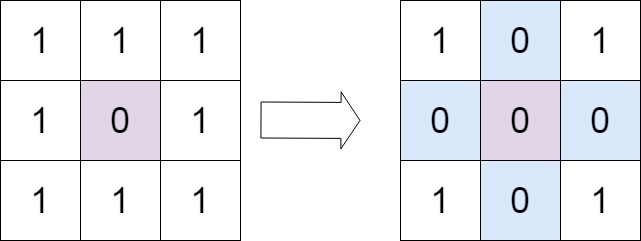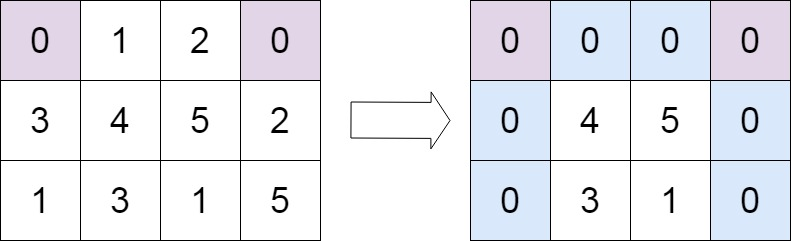# 73. Set Matrix Zeroes

Updated: 2023-09-01

LeetCode problem

Given an m x n integer matrix matrix, if an element is 0, set its entire row and column to 0’s.

You must do it in place.

Example 1:Input: matrix = [[1,1,1],[1,0,1],[1,1,1]]
Output: [[1,0,1],[0,0,0],[1,0,1]]


Example 2:Input: matrix = [[0,1,2,0],[3,4,5,2],[1,3,1,5]]
Output: [[0,0,0,0],[0,4,5,0],[0,3,1,0]]


Approach 1:

Idea:

LeetCode Submission

class Solution:
def setZeroes(self, matrix: List[List[int]]) -> None:

rows = len(matrix)
cols = len(matrix)

# 1. Check first row/column for zero's
first_row_has_zero = 0 in matrix
first_col_has_zero = 0 in list(zip(*matrix))

# 2. Check other cells in matrix and save info in the 1st row/col if cell has zero's
for i in range(1, rows):
for j in range(1, cols):
if matrix[i][j] == 0:
matrix[j] = 0 # 1st row
matrix[i] = 0 # 1st col

# 3. Loop again through first row/column and overwrite cells according to the data from 1st row/column
#    except the 1st row/col
for i in range(1, rows):
for j in range(1, cols):
if matrix[j] == 0 or matrix[i] == 0:
matrix[i][j] = 0

# Fill 0s for the 1st row if needed
if first_row_has_zero:
matrix =  * cols

# Fill 0s for the 1st col if needed
if first_col_has_zero:
for row in matrix:
row = 0